# Python中break/continue/pass的区别

Python中可以使用两种类型的循环：for循环和while循环。您可以将它们用于重复任务。因此，重复性任务将自动发生，使流程更加高效。不幸的是，您的循环可能会遇到一些问题。有时，您的程序可能会遇到问题，需要它跳过循环的一部分或完全退出循环。或者你需要它来忽略影响程序的外部因素。如果这是要添加到程序中的内容，则需要使用break、continue和pass语句。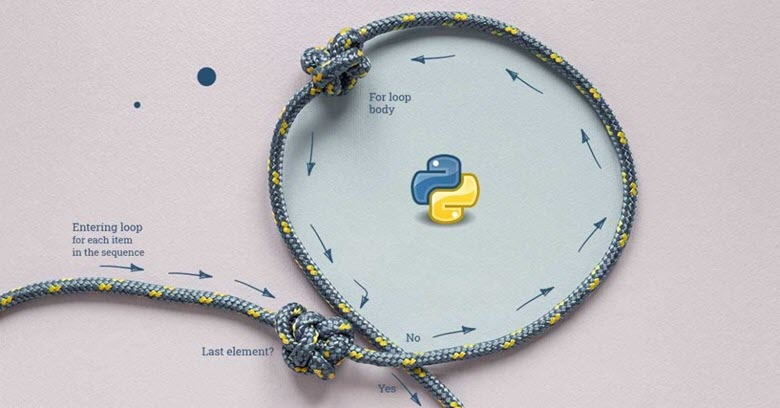## break

break语句负责终止使用它的循环。如果在嵌套循环中使用break语句，则当前循环将终止，并且流将继续执行循环后面的代码。

break语句的流程图：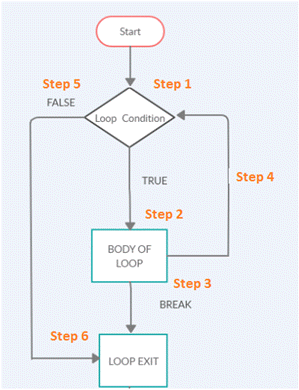• 步骤1）循环执行开始。
• 步骤2）如果循环条件为true，它将执行步骤2，其中将执行循环体。
• 步骤3）如果循环的主体具有break语句，则循环将退出并转至步骤6。
• 步骤4）循环条件执行并完成后，将进入步骤4中的下一个迭代。
• 步骤5）如果循环条件为false，它将退出循环并转至步骤6。
• 步骤6）循环结束。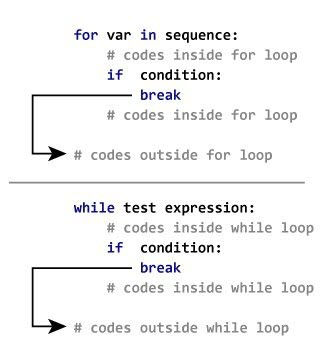while循环

my_list = ['Siya', 'Tiya', 'Guru', 'Daksh', 'Riya', 'Guru']
i = 0

while True:
print(my_list[i])
if my_list[i] == 'Guru':
print('Found the name Guru')
break
print('After break statement')
i += 1

print('After while-loop exit')


Siya
Tiya
Guru
Found name Guru
After while-loop exit


for循环

my_list = ['Siya', 'Tiya', 'Guru', 'Daksh', 'Riya', 'Guru']

for i in range(len(my_list)):
print(my_list[i])
if my_list[i] == 'Guru':
print('Found the name Guru')
break
print('After break statement')

print('Loop is Terminated')


Siya
Tiya
Guru
Found the name Guru
Loop is Terminated


for i in range(4):
for j in range(4):
if j==2:
break
print("The number is ",i,j);


The number is  0 0
The number is  0 1
The number is  1 0
The number is  1 1
The number is  2 0
The number is  2 1
The number is  3 0
The number is  3 1


Break只会跳出一层。

## continue

continue语句的流程图：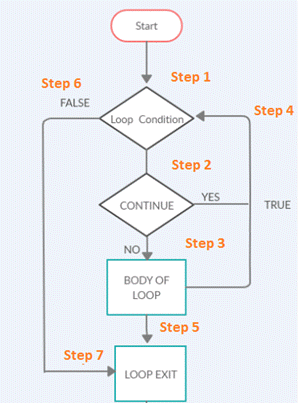• 步骤1）循环执行开始。
• 步骤2）循环内的代码执行将完成。如果循环中有一个continue语句，控件将返回到步骤4，即下一次迭代循环的开始。
• 步骤3）循环内的代码执行将完成。
• 步骤4）如果有一个continue语句，或者在主体内循环执行完成，它将调用下一个迭代。
• 步骤5）循环执行完成后，循环将退出并转至步骤7。
• 步骤6）如果步骤1中的循环条件失败，它将退出循环并转到步骤7。
• 步骤7）循环结束。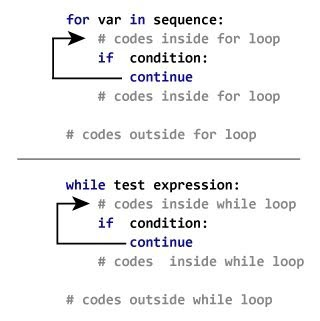while循环

i = 0
while i <= 10:
if i == 7:
i += 1
continue
print("The Number is  :" , i)
i += 1


The Number is  : 0
The Number is  : 1
The Number is  : 2
The Number is  : 3
The Number is  : 4
The Number is  : 5
The Number is  : 6
The Number is  : 8
The Number is  : 9
The Number is  : 10


for循环

for i in range(10):
if i == 7:
continue
print("The Number is :" , i)


The Number is : 0
The Number is : 1
The Number is : 2
The Number is : 3
The Number is : 4
The Number is : 5
The Number is : 6
The Number is : 8
The Number is : 9


for i in range(4):
for j in range(4):
if j==2:
continue
print("The number is ",i,j)


The number is  0 0
The number is  0 1
The number is  0 3
The number is  1 0
The number is  1 1
The number is  1 3
The number is  2 0
The number is  2 1
The number is  2 3
The number is  3 0
The number is  3 1
The number is  3 3


## pass

Python pass语句在循环、函数、类和if语句中用作占位符，不做任何事情，是一个空操作。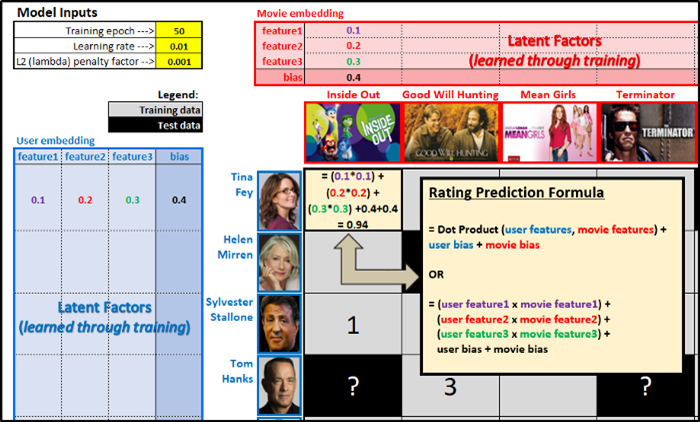##### 使用Excel搭建推荐系统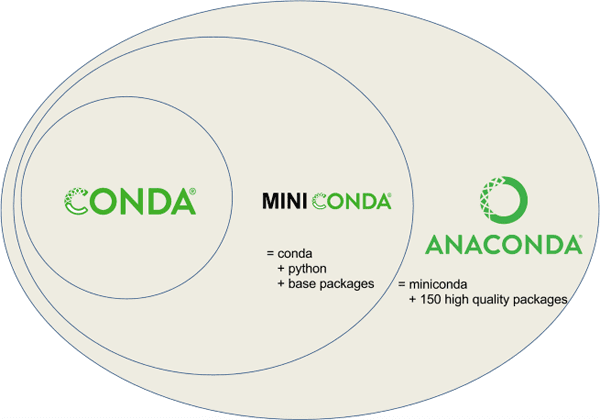##### Python数据科学IDE软件的选择